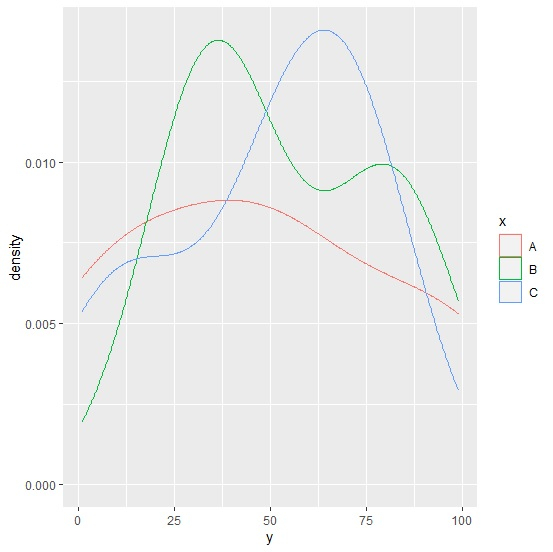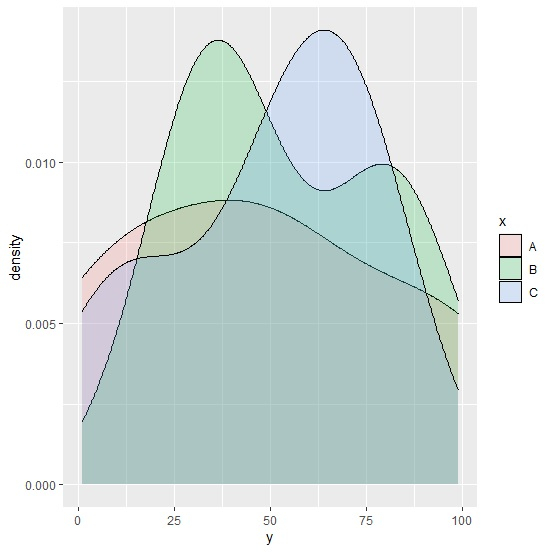# How to create density plot for categories filled with different colors in R?

To create density plot for categories filled with different colors, we can follow the below steps −

• Frist of all, create a data frame.
• Load ggplot2 package and creating the density plot for the categories.
• Create the density plot for the categories in the data frame by using fill function.

## Create the data frame

Let's create a data frame as shown below −

Live Demo

x<-sample(LETTERS[1:3],20,replace=TRUE)
y<-sample(1:100,20)
df<-data.frame(x,y)
df

On executing, the above script generates the below output(this output will vary on your system due to randomization) −

  x y
1 C 19
2 A 49
3 A 94
4 C 5
5 A 45
6 A 23
7 B 33
8 A 99
9 C 73
10 A 1
11 B 84
12 A 61
13 A 7
14 C 51
15 B 43
16 B 31
17 C 67
18 C 77
19 C 50
20 B 80

## Creating density plot for categories

Use geom_density with col function to create the density plot for categories −

library(ggplot2)
x<-sample(LETTERS[1:3],20,replace=TRUE)
y<-sample(1:100,20)
df<-data.frame(x,y)
ggplot(df,aes(y))+geom_density(aes(col=x),alpha=0.2)

### Output## Create the density plot for the categories filled with different colors

Use geom_density with fill function to create the density plot for categories filled with different colors −

x<-sample(LETTERS[1:3],20,replace=TRUE)
y<-sample(1:100,20)
df<-data.frame(x,y)
ggplot(df,aes(y))+geom_density(aes(fill=x),alpha=0.2)

### Output